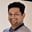Related Tags

java
lambda

# What are lambda expressions in Java?Tarun Telang

## Overview

We use a lambda expression as an anonymous function to pass around a value in Java. Lambda expressions are often used in conjunction with functional interfaces, which are interfaces that contain only a single abstract method.

Functional interfaces can be found in the java.util.function package, and some of the more useful ones include the Predicate, Function, and Consumer interfaces.

### Syntax

We write the lambda expressions in the following format:

(parameters) -> {body}
Syntax of a lambda expression

### Parameters

We can have multiple parameters, but the parameters of the lambda expression are optional. We separate multiple parameters with commas.

The body of the lambda expression contains the code that will be executed when the lambda expression is invoked.

For example, consider the following lambda expression:

(int x, int y) -> x + y
Example of lambda expression

This lambda expression takes in two parameters ($x$and $y$) and returns the sum of those two values.

### Example

To use a lambda expression with a functional interface, we can do as follows:

import java.util.function.*;

public class Main {
public static void main( String args[] ) {
adder = (x, y) -> x + y;

System.out.println(result);
}
}
Using a functional interface in Java

### Explanation

• Line 5: We use the BiFunction functional interface from the java.util.function package. The BiFunction interface is a generic interface that takes in three type parameters: the first two parameters are for specifying the type of the two input parameters, and the third parameter is for the type of the return value. In the above example, we're specifying that the first parameter is an Integer, the second parameter is an Integer, and the return value is also an Integer.
• Line 6: It will invoke the lambda expression, passing in the values 1 and 2 for the x and y parameters. The result of the lambda expression will be 3, which is what will be stored in the result variable.
• Line 8: We invoke the lambda expression by calling the apply() method on the BiFunction object adder .

As we can see, lambda expressions can be used to write code in a more concise and functional style.

RELATED TAGS

java
lambda

CONTRIBUTORTarun Telang
RELATED COURSES

View all Courses

Keep Exploring

Learn in-demand tech skills in half the time